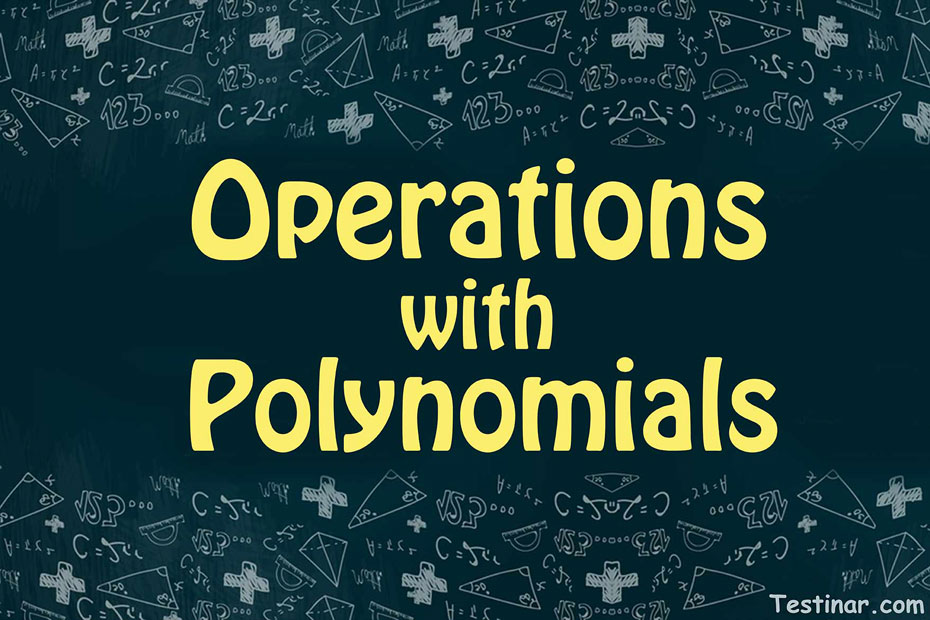## How to Do Operations with Polynomials

### How can we Define Polynomials?

To understand the proper definition of polynomials, we must break the word into two “poly” and “nomials”. This means a variety of expressions. To be extremely precise, a polynomial is an algebraic expression which contains various terms separated by certain mathematical operations. These terms can be pure variables, pure constants, or even mixed terms such as variables with coefficients. Now, a crucial point to determining a polynomial is that the variable in the expression (suppose $$x$$), should not have a fractional power.
For example, $$2x^{\frac{1}{3}} \ + \ 3x \ + \ 1$$ is not a polynomial as the first term has a fraction power of $$x$$.

### Types of Polynomials

Based on the number of terms, there are 3 different types of polynomials. They are monomials, binomials and trinomials. Let’s discuss more about them:

• Monomial: They consist of just a single term but there is one condition. The term cannot be zero. Ex: $$6x^2, \ 7x^3, 67x$$, etc.
• Binomial: Binomials consist of just two terms and they must have a mathematical operation in between. Ex: $$6x^2 \ + \ 7x^3, \ 8x^2 \ + \ 7y, \ xy^2 \ + \ 3x$$, etc.
• Trinomial: Trinomial consist of three terms and between every two terms there is a mathematical operation. Ex: $$2x^2 \ + \ 3x \ + \ 10, \ 4x^3 \ + \ 3x^2 \ + \ 7, \ 3x^2y \ + \ 4xy \ + \ 6$$, etc.

### What are Like and Unlike Terms?

In algebra, we have the concept of like and unlike terms. So, if in an expression, you find that two or more same variables have the same exponential powers, then they are considered like terms. So, what we can do to simplify the expression is club those like terms by performing basic mathematical operations between them.
Also, in the case of unlike terms, you will find that the exponential powers of two or more same variables are not the same. So, we have no other option other than to leave them as they are.
Some examples of like terms are $$2x^3 \ - \ x^3, \ 7x^2 \ + \ 3x^2$$. Here we can see that the power of $$x$$ is the same in both the examples. Also, some examples of unlike term expressions would be $$7x^2 \ - \ 9x^3, \ -x^3 \ + \ x^2$$.

### Operations with Polynomials

When operating with polynomial expression, apply the below-mentioned steps:

• First, simplify the expression by adding/subtracting the like terms. Also. Don’t forget to apply the PEMDAS rule in case of brackets or parenthesis.
• Also, wherever possible, use the distributive property.

Ex: $$4x^3 \ + \ 3x^3 \ + \ 2x^2 \ - \ x^2 \ + \ 9 \ = \ 7x^3 \ + \ x^2 \ + \ 9$$.

## Operations with Polynomials Practice Quiz

### The Most Comprehensive CHSPE Math Preparation Bundle

$76.99$36.99

### ASVAB Math Full Study Guide

$25.99$13.99

### ATI TEAS 6 Math in 10 Days

$20.99$19.99

### Accuplacer Math Workbook

$21.99$15.99